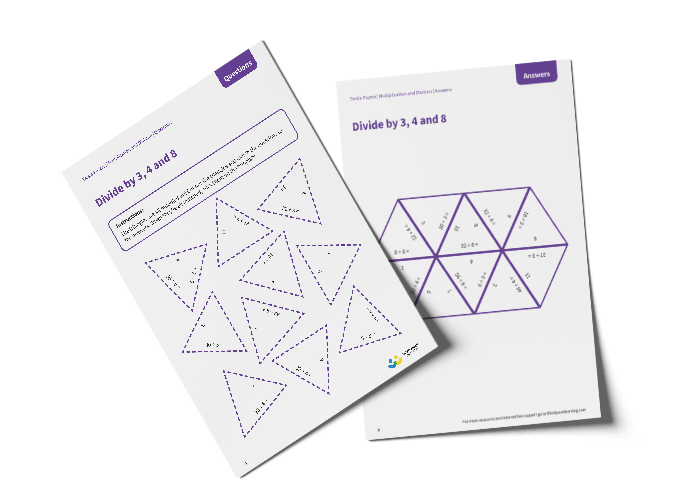# Tarsia Puzzle Divide by 3, 4 and 8 (Year 3)

The triangles in the tarsia puzzle have all been mixed up. In this dividing by 3, 4 and 8 tarsia puzzle, pupils will need to match the division question to the correct answer to create one large hexagon.

This Year 3 divide by 3, 4 and 8 tarsia puzzle worksheet contains:

• One question sheet
• One template sheet

Year 3

Division